# Some notes on PChange – estimating when trajectories cross over time

J.C. Barnes and company published a paper in JQC not too long ago and came up with a metric, PChange, to establish the number of times trajectories cross in a sample. This is more of interest to life course folks, although it is not totally far fetched to see it applied to trajectories of crime at places. Part of my interest in it was simply that it is an interesting statistical question — when two trajectories with errors cross. A seemingly simple question that has a few twists and turns. Here are my subsequent notes on that metric.

# The Domain Matters

First, here is an example of the trajectories not crossing: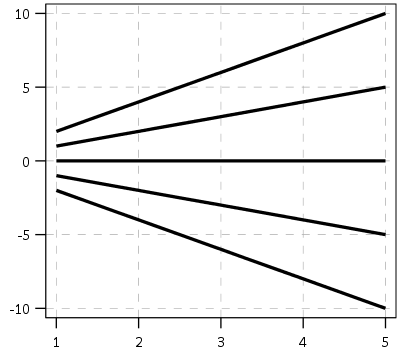This points to an important assumption about those lines not crossing though that was never mentioned in the Barnes paper — the domain matters. For instance, if we draw those rays further back in time what happens?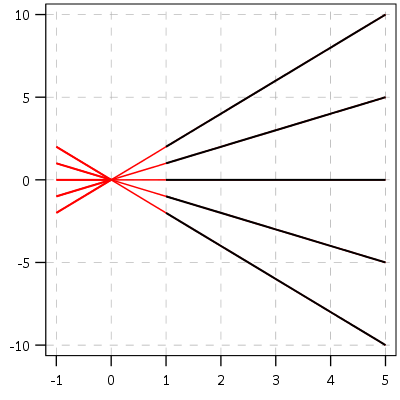They cross! This points to an important piece of information when evaluating PChange — the temporal domain in which you examine the data matters. So if you have a sample of juvenile delinquency measures from 14-18 you would find less change than a similar sample from 12-20.

This isn’t really a critique of PChange — it is totally reasonable to only want to examine changes within a specific domain. Who cares if delinquency trajectories cross when people are babies! But it should be an important piece of information researchers use in the future if they use PChange — longer samples will show more change. It also won’t be fair to compare PChange for samples of different lengths.

# A Functional Approach to PChange

For above you may ask — how would you tell if a trajectory crosses outside of the domain of the data? The answer to that question is you estimate an underlying function of the trajectory — some type of model where the outcome is a function of age (or time). With that function you can estimate the trajectory going back in time or forward in time (or in between sampled measurements). You may not want to rely on data outside of the domain (its standard error will be much higher than data within the time domain, forecasting is always fraught with peril!), but the domain of your sample is ultimately arbitrary. So what about the question will the trajectories ever cross? Or would the trajectories have crossed if I had data for ages 12-20 instead of just 16-18? Or would they have crossed if I checked the juveniles at age 16 1/2 instead of only at 16?

So actually instead of the original way the Barnes paper formulated PChange, here is how I thought about calculating PChange. First you estimate the underlying trajectory for each individual in your sample, then you take the difference of those trajectories.

``````y_i = f(t)
y_j = g(t)
y_delta = f(t) - g(t) = d(t)``````

Where `y_i` is the outcome `y` for observation `i`, and `y_j` is the outcome `y` for observation `j`. `t` is a measure of time, and thus the anonymous functions `f` and `g` represent growth models for observations i and j over time. `y_delta` is then the difference between these two functions, which I represent as the new function `d(t)`. So for example the functions for each individual might be quadratic in time:

``````y_i = b0i + b1i(t) + b2i(t^2)
y_j = b0j + b1j(t) + b2j(t^2)``````

Subsequently the difference function will also be quadratic, and can be simply represented as:

``y_delta = (b0i - b0j) + (b1i - b1j)*t + (b2i - b2j)*t^2``

Then for the trajectories to cross (or at least touch), `y_delta` just then has to equal zero at some point along the function. If this were math, and the trajectories had no errors, you would just set d(t) = 0 and solve for the roots of the equation. (Most people estimating models like these use functions that do have roots, like polynomials or splines). If you cared about setting the domain, you would then just check if the roots are within the domain of interest — if they are, the trajectories cross, if they are not, then they do not cross. For data on humans with age, obviously roots for negative human years will not be of interest. But that is a simple way to solve the domain problem – if you have an underlying estimate of the trajectory, just see how often the trajectories cross within equivalent temporal domains in different samples.

I’d note that the idea of having some estimate of the underlying trajectory is still relevant even within the domain of the data — not just extrapolating to time periods outside. Consider two simple curves below, where the points represent the time points where each individual was measured.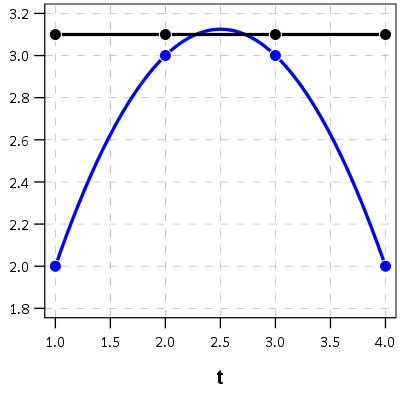So while the two functions cross, when only considering the sampled locations, like is done in Barnes et al.’s PChange, you would say these trajectories do not cross, when in actuality they do. It is just the sampled locations are not at the critical point in the example for these two trajectories.

This points to another piece of contextual information important to interpreting PChange — the number of sample points matter. If you have samples every 6 months, you will likely find more changes than if you just had samples every year.

I don’t mean here to bag on Barnes original metric too much — his PChange metric does not rely on estimating the underlying functional form, and so is a non-parametric approach to identifying change. But estimating the functional form for each individual has some additional niceties — one is that you do not need the measures to be at equivalent sample locations. You can compare someone measured at 11, 13, and 18 to someone who is measured at 12, 16, and 19. For people analyzing stuff for really young kids I bet this is a major point — the underlying function at a specific age is more important then when you conveniently measured the outcome. For older kids though I imagine just comparing the 12 to 11 year old (but in the same class) is probably not a big deal for delinquency. It does make it easier though to compare say different cohorts in which the measures are not at nice regular intervals (e.g. Add Health, NLYS, or anytime you have missing observations in a longitudinal survey).

In the end you would only want to estimate an underlying functional form if you have many measures (more so than 3 in my example), but this typically ties in nicely with what people modeling the behavior over time are already doing — modeling the growth trajectories using some type of functional form, whether it is a random effects model or a group based trajectory etc., they give you an underlying functional form. If you are willing to assume that model is good enough to model the trajectories over time, you should think it is good enough to calculate PChange!

# The Null Matters

So this so far would be fine and dandy if we had perfect estimates of the underlying trajectories. We don’t though, so you may ask, even though `y_delta` does not exactly equal zero anywhere, its error bars might be quite wide. Wouldn’t we then still infer that there is a high probability the two trajectories cross? This points to another hidden assumption of Barnes PChange — the null matters. In the original PChange the null is that the two trajectories do not cross — you need a sufficient change in consecutive time periods relative to the standard error to conclude they cross. If the standard error is high, you won’t consider the lines to cross. Consider the simple table below:

``````Period A_Level A_SE B_Level B_SE
1      4         1    1.5   0.5
2      5         1    3     0.5
3      6         1    4.5   0.5
4      7         1    6     0.5``````

Where A_Level and B_Level refer to the outcome for the four time periods, and A_SE and B_SE refer to the standard errors of those measurements. Here is the graph of those two trajectories, with the standard error drawn as areas for the two functions (only plus minus one standard error for each line).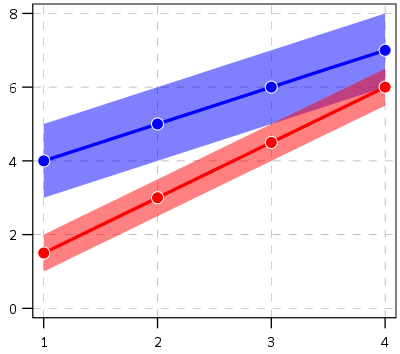And here is the graph of the differences — assuming that the covariance between the two functions is zero (so the standard error of the difference equals `sqrt(A_SE^2 + B_SE^2)`). Again only plus/minus one standard error.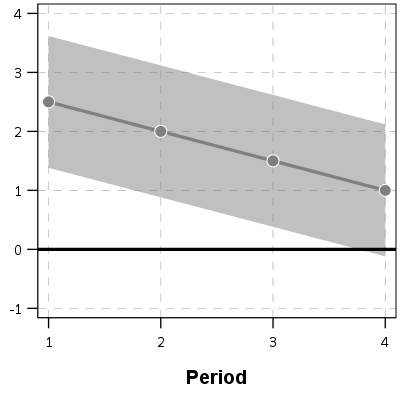You can see that the line never crosses zero, but the standard error area does. If our null is `H0: y_delta = 0 for any t`, then we would fail to reject the null in this example. So in Barnes original PChange these examples lines would not cross, whereas with my functional approach we don’t have enough data to know they don’t cross. This I suspect would make a big difference in many samples, as the standard error is going to be quite large unless you have very many observations and/or very many time points.

If one just wants a measure of crossed or did not cross, with my functional approach you could set how wide you want to draw your error bars, and then estimate whether the high or low parts of that bar cross zero. You may not want a discrete measure though, but a probability. To get that you would integrate the probability over the domain of interest and calculate the chunk of the function that cross zero. (Just assume the temporal domain is uniform across time.)

So in 3d, my difference function would look like this, whereas to the bottom of the wall is the area to calculate the probability of the lines crossing, and the height of the surface plot is the PDF at that point. (Note the area of the density is not normalized to sum to 1 in this plot.)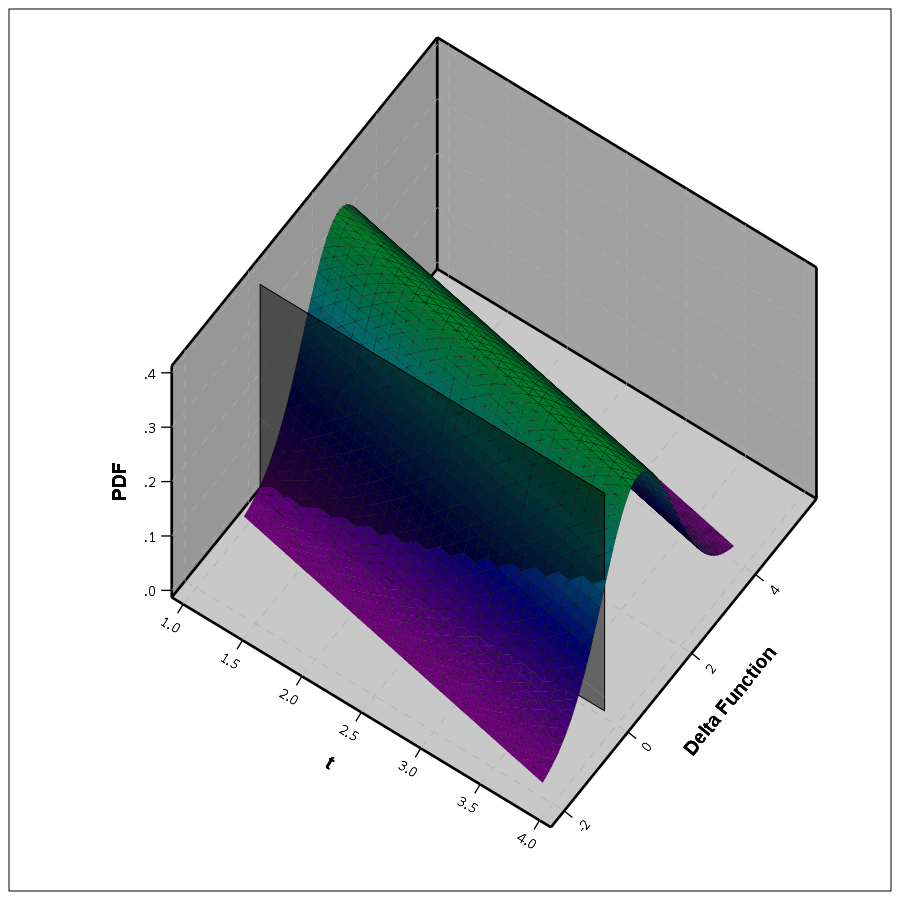This surface graph ends up crossing more than was observed in my prior 2d plots, as I was only plotting 1 standard error. Here imagine that the top green part of the density is the mean function — which does not cross zero — but then you have a non-trivial amount of the predicted density that does cross the zero line.

In the example where it just crosses one time by a little, it seems obvious to consider the small slice as the probability of the two lines crossing. I think to extend this to not knowing to test above or below the line you could calculate the probability on either side of the line, take the minimum, and then double that minimum for your p-value. So if say 5% of the area is below the line in my above example, you would double it and say the two-tailed p-value of the lines crossing is p = 0.10. Imagine the situation in which the line mostly hovers around 0, so the mass is about half on one side and half on the other. In that case the probability the lines cross seems much higher than 50%, so doubling seems intuitively reasonable.

So if you consider this probability to be a p-value, with a very small p-value you would reject the null that the lines cross. Unlike most reference distributions for p-values though, you can get a zero probability estimate of the lines crossing. You can aggregate up those probabilities as weights when calculating the overall PChange for the sample. So you may not know for certain if two trajectories cross, but you may be able to say these two trajectories cross with a 30% probability.

Again this isn’t to say that PChange is bad — it is just different. I can’t give any reasoning whether assuming they do cross (my functional approach) or assuming they don’t cross (Barnes PChange) is better – they are just different, but would likely make a large difference in the estimated number of crossings.

# Population Change vs Individual Level Change

So far I have just talked about trying to determine whether two individual lines cross. For my geographic analysis of trajectories in which I have the whole population (just a sample in time), this may be sufficient. You can calculate all pairwise differences and then calculate PChange (I see no data based reason to use the permutation sample approach Barnes suggested – we don’t have that big of samples, we can just calculate all pairwise combinations.)

But for many of the life course researchers, they are more likely to be interested in estimating the population of changes from the samples. Here I will show how you can do that for either random effects models, or for group based trajectory models based on the summary information. This takes PChange from a sample metric to a population level metric implied via your models you have estimated. This I imagine will be much easier to generalize across samples than the individual change metrics, which will be quite susceptible to outlier trajectories, especially in small samples.

First lets start with the random effects model. Imagine that you fit a linear growth model — say the random intercept has a variance of 2, and the random slope has a variance of 1. So these are population level metrics. The fixed effects and the covariance between the two random effect terms will be immaterial for this part, as I will discuss in a moment.

First, trivially, if you selected two random individuals from the population with this random effects distribution, the probability their underlying trajectories cross at some point is 1. The reason is for linear models, two lines only never cross if the slopes are perfectly parallel. Which sampling from a continuous random distribution has zero probability of them being exactly the same. This does not generalize to more complicated functions (imagine parabolas concave up and concave down that are shifted up and down so they never cross), but should be enough of a motivation to make the question only relevant for a specified domain of time.

So lets say that we are evaluating the trajectories over the range `t = [10,20]`. What is the probability two individuals randomly sampled from the population will cross? So again with my functional difference approach, we have

``````y_i = b0i + b1i*t
y_j = b0j + b1j*t
y_delta = (b0i - b0j) + (b1i - b1j)*t``````

Where in this case the `b0` and `b1` have prespecified distributions, so we know the distribution of the difference. Note that in the case with no covariates, the fixed effects will cancel out when taking the differences. (Generalizing to covariates is not as straightforward, you could either assume they are equal so they cancel out, or you could have them vary according to additional distributions, e.g. males have an 90% chance of being drawn versus females have a 10% chance, in that case the fixed effects would not cancel out.) Here I am just assuming they cancel out. Additionally, taking the difference in the trajectories also cancels out the covariance term, so you can assume the covariance between `(b0i - b0j)` and `(b1i - b1j)` is zero even if `b0` and `b1` have a non-zero covariance for the overall model. (Post is long enough — I leave that as an exercise for the reader.)

For each of the differences the means will be zero, and the variance will be the two variances added together, e.g. `b0i - b0j` will have a mean of zero and a variance of `2 + 2 = 4`. The variance of the difference in slopes will then be 2. Now to figure out when the two lines will cross.

If you make a graph where the X axis is the difference in the intercepts, and the Y axis is the difference in the slopes, you can then mark off areas that indicate the two lines will cross given the domain. Here for example is a sampling of where the lines cross – red is crossing, grey is not crossing.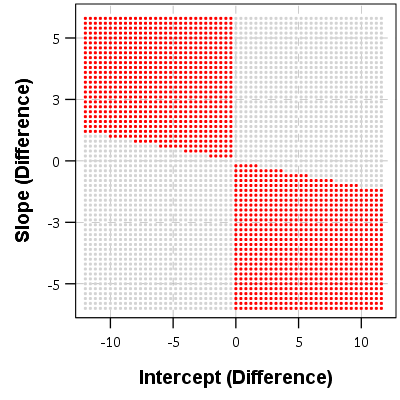So for example, say we had two random draws:

``````y_i = 1   + 0.5*t
y_j = 0.5 + 0.3*t
y_delta = 0.5 + 0.2*t``````

This then shows that the two lines do not cross when only evaluating `t` between 10 and 20. They have already diverged that far out (you would need negative `t` to have the lines cross). Imagine if `y_delta = -6 + 0.2*t` though, this line does cross zero though, at `t = 10` this function equals -1, whereas at `t = 20` the function equals 4.

If you do another 3d plot you can plot the bivariate PDF. Again integrate the chunks of areas in which the function crosses zero, and voila, you get your population estimate.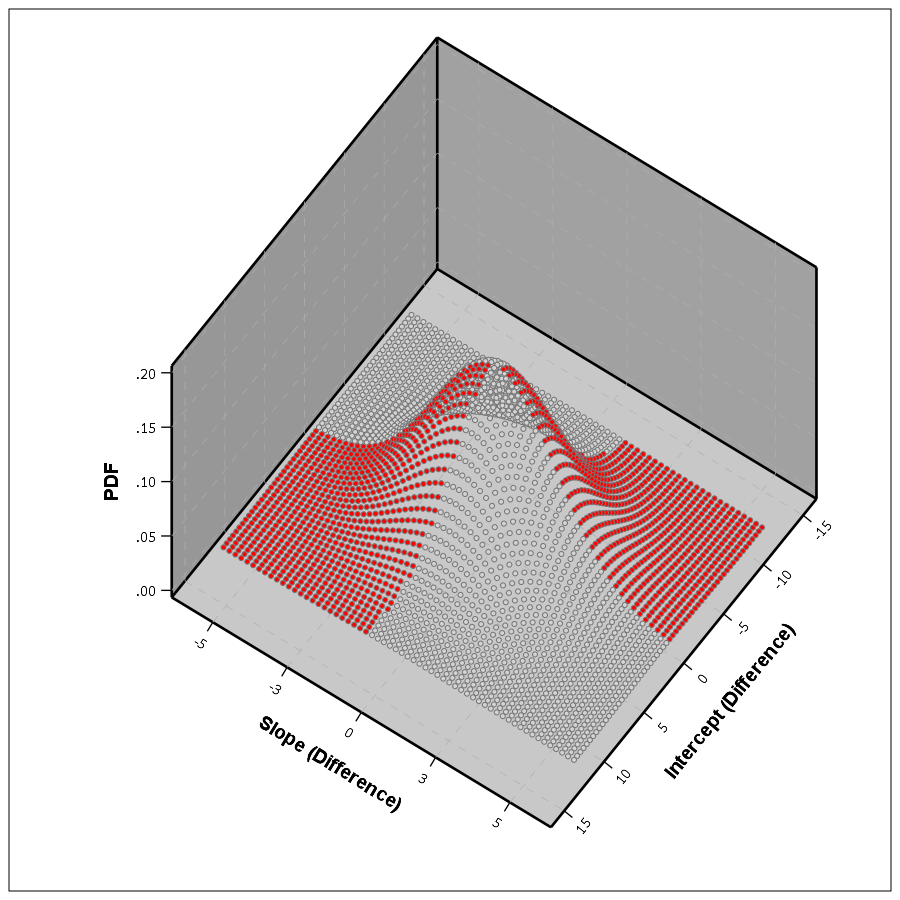This works in a similar manner to higher order polynomials, but you can’t draw it in a nice graph though. I’m blanking at the moment of a way to find these areas offhand in a nice way — suggestions welcome!

This gets a bit tricky thinking about in relation to individual level change. This approach does not assume any error in the random draws of the line, but assumes the draws will have a particular distribution. So the PChange does not come from adding up whether individual lines in your sample cross, it comes from the estimated distribution of what the difference in two randomly drawn lines would look like that is implied by your random effects model. Think if based on your random effect distribution you randomly drew two lines, calculated if they crossed, and then did this simulation a very large number of times. The integrations I’m suggesting are just an exact way to calculate PChange instead of the simulation approach.

If you were to do individual change from your random effects model you would incorporate the standard error of the estimated slope and intercept for the individual observation. This is for your hypothetical population though, so I see no need to incorporate any error.

Estimating population level change from group based trajectory models via my functional approach is more straightforward. First, with my functional approach you would assume individuals who share the same latent trajectory will cross with a high probability, no need to test that. Second, for testing whether two individual trajectories cross you would use the approach I’ve already discussed around individual lines and gain the p-value I mentioned.

So for example, say you had a probability of 25% that a randomly drawn person from group A would cross a randomly drawn person from Group B. Say also that Group A has 40/100 of the sample, and Group B is 60/100. So then we have three different groups: A to A, B to B, and A to B. You can then break down the pairwise number in each group, as well as the number of crosses below.

``````Compare   N    %Cross Cross
A-A      780    100    780
B-B     1770    100   1770
A-B     2400     25    600
Total   4950     64   3150``````

So then we have a population level p-change estimate of 64% from our GBTM. All of these calculations can be extended to non-integers, I just made them integers here to simplify the presentation.

Now, that overall PChange estimate may not be real meaningful for GBTM, as the denominator includes pairwise combinations of folks in the same trajectory group, which is not going to be of much interest. But just looking at the individual group solutions and seeing what is the probability they cross could be more informative. For example, although Barnes shows the GBTM models in the paper as not crossing, depending on how wide the standard errors of the functions are (that aren’t reported), this functional approach would probably assign non-zero probability of them crossing (think low standard error for the higher group crossing a high standard error for the low group).

Phew — that was a long post! Let me know in the comments if you have any thoughts/concerns on what I wrote. Simple question — whether two lines cross — not a real simple solution when considering the statistical nature of the question though. I can’t be the only person to think about this though — if you know of similar or different approaches to testing whether two lines cross please let me know in the comments.